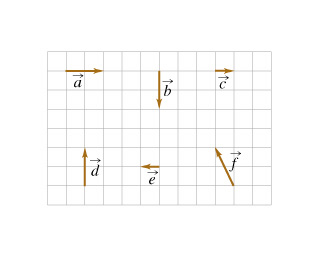# Problem: Six vectors (a through f ) have the magnitudes and directions indicated in the figure. (Figure 1)Rank the vector combinations on the basis of their magnitude. Rank from largest to smallest. To rank items as equivalent, overlap them.a+cf+cda+ba+ea+d

###### FREE Expert Solution

2D vector magnitude:

$\overline{)\mathbf{|}\stackrel{\mathbf{⇀}}{\mathbf{r}}\mathbf{|}{\mathbf{=}}\sqrt{{\mathbf{x}}^{\mathbf{2}}\mathbf{+}{\mathbf{y}}^{\mathbf{2}}}}$

a + c = 2i + 1i = 3i

|a + c| = 3

f + c = (-1i + 2j) + 1i = 2j

|f + c| = 2

d = 2j

94% (39 ratings)###### Problem Details

Six vectors (a through f ) have the magnitudes and directions indicated in the figure. (Figure 1)Rank the vector combinations on the basis of their magnitude.

Rank from largest to smallest. To rank items as equivalent, overlap them.

a+c

f+c

d

a+b

a+e

a+d

Frequently Asked Questions

What scientific concept do you need to know in order to solve this problem?

Our tutors have indicated that to solve this problem you will need to apply the Adding Vectors Graphically concept. You can view video lessons to learn Adding Vectors Graphically. Or if you need more Adding Vectors Graphically practice, you can also practice Adding Vectors Graphically practice problems.